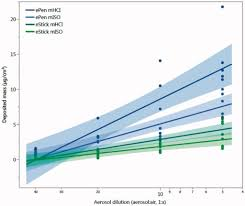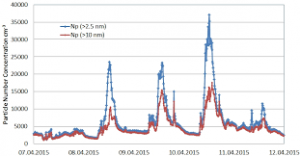## How to Calculate and Solve for the Molar Concentration, Molar Mass and Mass Concentration in Chemistry | The Calculator EncyclopediaThe image above represents the mass concentration.

To compute for mass concentration, two essential parameters are needed and these are molar concentration (c) and molar mass (M).

ρ = c x M

Where;
ρ = mass concentration
c = molar concentration
M = molar mass

Let’s solve an example;
Find the mass concentration when the molar concentration is 24 and molar mass is 15.

This implies that;
c = molar concentration = 24
M = molar mass = 15

ρ = c x M
ρ = 24 x 15
ρ = 360

Therefore, the mass concentration is 360 Kg/dm³.

Calculating the Molar Concentration when the Mass concentration and Molar Mass.

c = ρ / M

Where;
c = molar concentration
ρ = mass concentration
M = molar mass

Let’s solve an example;
Find the molar concentration when the mass concentration is 120 with a molar mass of 40.

This implies that;
ρ = mass concentration = 120
M = molar mass = 40

c = ρ / M
c = 120 / 40
c = 3

Therefore, the molar concentration is 3 mol/L.

## How to Calculate and Solve for the Mass, Volume and Mass Concentration in Chemistry | The Calculator EncyclopediaThe image above represents the mass concentration.

To compute the mass concentration, two essential parameters are needed and these parameters are mass (m) and volume (V).

The formula for calculating mass concentration:

ρ = mV

Where;
ρ = Mass concentration
m = Mass
V = Volume

Let’s solve an example;
Find the mass concentration when the mass is 8 kg with a volume of 24 dm³.

This implies that;
m = Mass = 8
V = Volume = 24

ρ = mV
ρ = 824
ρ = 0.33

Therefore, the mass concentration is 0.33 Kg/dm³.

Calculating the Mass when the Mass Concentration and the Volume is Given.

m = Vρ

Where;
m = Mass
ρ = Mass concentration
V = Volume

Let’s solve an example;
Find the mass when the mass concentration is 12 kg/dm³ with a volume of 7 dm³.

This implies that;
ρ = Mass concentration = 12
V = Volume = 7

m = Vρ
m = 12 x 7
m = 84

Therefore, the mass concentration is 84 kg.